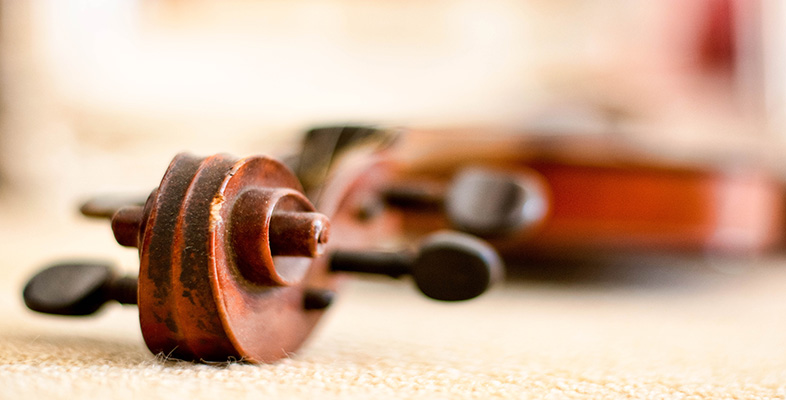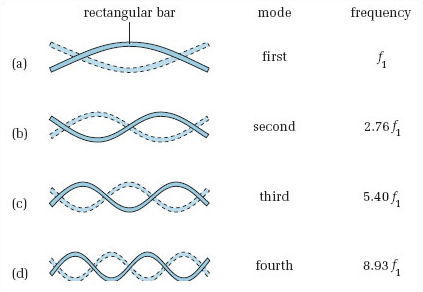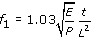Creating musical sounds

Start this free course now. Just create an account and sign in. Enrol and complete the course for a free statement of participation or digital badge if available.

Free course

# 5.13.1 Rectangular bar

If a solid rectangular bar is excited by striking it, energy is supplied that starts the bar vibrating transversely. The bar will vibrate in a number of modes simultaneously since the striking action supplies energy over a range of frequencies. The motion of the bar will be the superposition of the standing-wave patterns of the excited modes.

Assume for the moment that the rectangular bar is supported in such a way that both ends are free to vibrate and the effects of the supports can be neglected. The standing-wave patterns for the bar are given in Figure 19. Note that for each normal mode of vibration, the ends of the bar are displacement antinodes (points of maximum vibration).

The fundamental vibrational mode is shown in Figure 19(a). In addition to the antinodes at the free ends, there is a third antinode at the centre of the bar and two nodes approximately halfway between the centre and each end. When the middle of the bar rises the ends fall, and vice versa. Each successive mode of vibration (Figure 19(b), (c) and (d)) has one additional displacement node and one additional antinode.Figure 19 The first four modes of vibration of a rectangular bar. The solid and dashed lines indicate the positions of the bar at opposite phase positions in the cycle

This is more complicated than the cases we have met before. For a start, the frequency of the first mode of vibration depends on a number of factors to do with the bar's size and what it's made of. In fact, the first natural frequency of the bar is given approximately by:where E is known as the Young's modulus and is a measure of the elasticity or rigidity of the material from which the bar is made, ρ is the density of the material, t is the thickness of the bar and L is the length of the bar. The Young's modulus is expressed in newtons per metre squared (N/m2), the density is expressed in kilograms per metre cubed (kg/m3) and the thickness and length are expressed in metres (m).

Looking at Figure 19, it would seem that once the frequency of the first mode of vibration has been determined from the above formula, the frequencies of the higher modes would form a harmonic series. This is because the wavelengths of the standing waves are related and look very similar to the situation for air vibrating in an air column that is open at both ends (you may like to refer back to Figure 15). However, frequency is related not only to wavelength but to wave speed. In the case of the rectangular bar it turns out that wave speed is not constant but increases with frequency (unlike a stretched string where the wave speed is essentially constant). Thus the frequencies of the increasing modes of vibration are spaced further apart than would be the case if wave speed was constant. The frequencies of the second, third and fourth modes of vibration actually turn out to be 2.76f1, 5.40f1 and 8.93f1. Don't worry, you don't need to memorise either these frequencies or the expression for the first natural frequency given above. The important thing that you need to note is that the frequencies are not harmonically related. That is, they do not form a harmonic series.

TA212_2